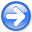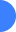Implementing implicit finite-difference in the time-space domain using spectral factorization and helical deconvolutionNext: Introduction Up: Reproducible Documents

### Abstract:

The method of modeling wavefield propagation with an implicit finite-difference approximation to the two-way acoustic isotropic wave equation, using spectral factorization and helical deconvolution, exhibits instability of the propagating wavefield as the time step is increased. In this study, I test several potential sources of the instability problem: the implicit finite-difference scheme itself, the precision of the floating point representation of the filter coefficients, the number of filter coefficients, and the spectral factorization method. None of these issues is the cause for the apparent instability.

2010-11-26